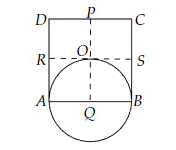# Prove that the circle drawn with any side of a rhombus as diameter, passes through the point of intersection of its diagonals.

To do:

We have to prove that the circle drawn with any side of a rhombus as diameter, passes through the point of intersection of its diagonals.

Solution:Draw a circle with $Q$ as the centre.

The circle passes through $A, B$ and $O$ as shown in the figure.

This implies,

$QA = QB = QO$     (Radii of the circle)

We know that,

All the sides of a rhombus are equal.

This implies,

$AB = CD$

Multiplying by $\frac{1}{2}$ on both sides, we get,

$\frac{1}{2}AB = \frac{1}{2}CD$

$\Rightarrow AQ = DP$

$BQ = DP$

$Q$ is the midpoint of $AB$

This implies,

$AQ= BQ$.........(i)

Similarly,

$RA = SB$

Draw $PQ$ parallel to $AD$

$RA = QO$.........(ii)

From (i) and (ii), we get,

$QA = QB = QO$

Hence proved.

Updated on: 10-Oct-2022

35 Views